THE CODE BEARING POSITIONS OF THE STANDING STONES

Of the estimated 60 standing stones that were originally erected at Ring o' Brodgar, only about 27 remain standing. These were mostly re-erected in 1906 and, in addition to them, another 13 or so stumps of broken stones remain. Future archaeological investigations should reveal with accuracy the former positions of the now missing, buried or broken stones and sockets, sufficient to extract the intended, original codes related to each component position. Let's now consider the codes inherent in the majority of stones that have survived, as distinguishable within Google Earth.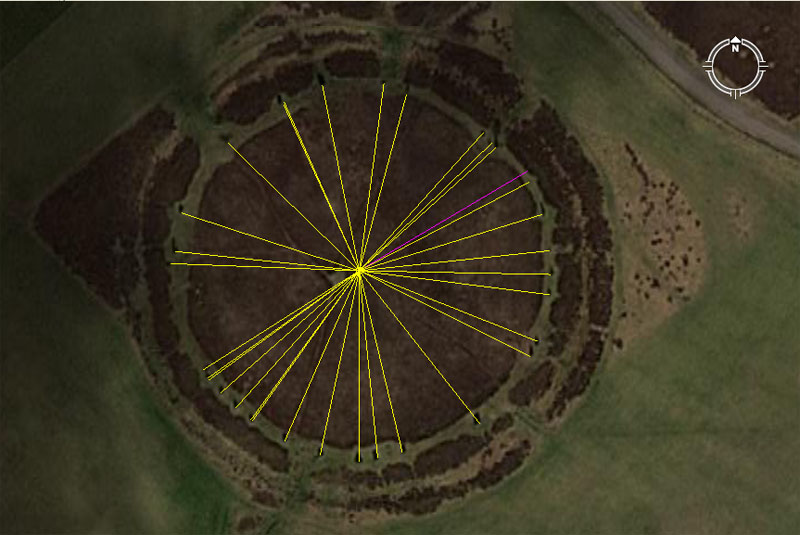The majority of what's left of the Ring o' Brodgar of standing stones can be seen in this Google Earth image. In 1906 many of these stones were stood up again and placed back into the socket troughs where they had originally stood. We can, therefore, proceed with confidence that the stones are at or very near to where the ancient builders of the ring originally stood them.

Let's now test each apparent position to see if any recurring distance and angle codes, found on other sites around Britain, can also be detected at Ring o' Brodgar.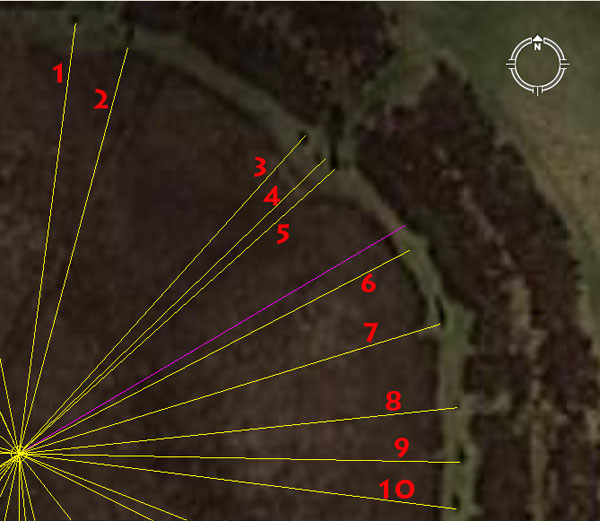1. This stone sits 170.1' out @ 7.5-degrees (187.5-degrees return).

This radius code relates to the lunar nutation cycle of 6804-days, which is the Moon's equivalent to the Sun moving to the furthermost extremities of the horizon, from Summer to Winter Solstice and back again. The Moon does a similar thing, except it's over a much longer time (18.613-years .... lunar minor standstill to lunar major standstill, then back again to minor standstill again). The actual time it takes is 6798.36-days, but that value isn't factorable, so the ancient mathematician-astronomers accepted a miniscule amount of error in order to be able to describe the long cycle using a factorable number (6804-days ...about five days too many). The use of a close-proximity factorable number meant, however, that ancient mathematicians could calculate the Moon's cycle down to days, hours, minutes or seconds in time and describe it in a lunisolar Sabbatical Calendar system, to a tolerance of less than a minute of error in a lunar month.

The sum of 1701-days would be 1/4th of the 6804-day cycle. The diameters of both Brodgar Ring and Avebury Henge's southern circle were designed to convey 340.2' and, in days, that value would equate to 1/20th of the 6804-day period. The inch value for a complete circuit around the base of the Khafre Pyramid of Egypt @ 708.75' per side was 34020".

The angle value of 7.5-degrees generates a mathematical progression that was very important to compass calibrations, as well as navigation by the Greek mile of 5250'.

The return angle of 187.5 functions in much the same way within a mathematical progression and generates very important compass calibration, lunar and navigational numbers. The sum of 187.5' = 1/28th of a Greek mile.

2. This stone sits 165' out @ 15-degrees.

The distance is 10 rods or perches of 16.5' each (3 ancient fathoms). This distance is also 1/100th of an ancient English league (16500' or 3.125-miles) or 1/32nd of an English mile (5280') or 1/36th of an ancient Scottish mile (5940').

The 15 value sets up a mathematical progression that is very useful in compass readings and 15-degrees is 1/24th of a 360-degree circle.

3. This stone sits 168' out @ 42-degrees.

The sum of 168' is 1/40th of an ancient Irish mile of 6720'. It sets up a mathematical progression that was very important to navigation.

The entire ("6&7") "Greek-mile" system of length is dependant upon the value "42" (6 X 7) as its base increment, as is the Irish system.

4. This stone sits 166.66666' out @ 46.08-degrees.

In any 3,4,5 triangle the length of the shortest side will be 1.6666666 less than the hypotenuse (long side).

A mathematical progression based upon 46.08 will generate numbers much used in navigation.

5. This stone sits 166.66666' out @ 48-degrees.

The 48 value is essential to many kinds of calculations and 480' is 1/11th of an English mile of 5280' or 1/14th of an Irish mile of 6720'.

5a. (Purple line). According to a map plan, a stone should repose on this line, but can't be visually detected. The angle shown, which might represent the position, is 59.4-degrees.

6. This stone sits 172.8' out @ 62.5-degrees.

The value 1728 (12 X 12 X 12) is very important to ancient calculations of volume or navigation. A cubic foot is 1728 cubic inches.

The 62.5 value relates equally to navigation and the cycle of the Moon and sets up a mathematical progression useful to both.

7. This stone sits 172.8 out @ 72.9-degrees.

The value, 729 was used in lunar calculations and also in navigation using the Roman mile. The sum of 7.29" was 1/8th of a Roman pace of 58.32" (5 Roman feet of 11.664"). The Bush Barrow Lozenge artefact, found adjacent to Stonehenge, has a design length of 7.29".

8. This stone sits 172.8-degrees out @ 264-degrees return.

The sum of 2640' is 1/2 an English mile of 5280'.

9. This stone sits 172.8' out @ 91.125-degrees.

This is, essentially the same as the 729, lunar progression, except at a more refined base division. There would be 28 intervals of 91.125-days in the 2551.5-day period (7.2 lunar years) monitored within the lunisolar Sabbatical Calendar.

10. This stone sits 172.8' out @ 97.2-degrees.

The importance of this stone cannot be overemphasised as the vector crossed the Ring o' Brodgar stone then resolved upon the highest point in the Orkney Islands, the summit of Wideford Hill at Kirkwall (7.5 Greek miles away). This Ring o' Brodgar stone was, therefore a trig-fix position, tied to an eternally present and immovable geological feature in the Orkney Island's landscape. The reason why the centre of Ring o Brodgar was placed at that precise position related directly to the position of Wideford Hill's summit (ESE), simultaneously to the epicentre of Hoy Island's "V" trough between Wade Hill & Cuilags Hill (SSW).

The value 97.2 is part of a navigational & lunar progression simultaneously and the sum of 97.2-miles would be 1/256th of the 24883.2-mile equatorial circumference, whereas 972-days would be 1/7th of the 6804-day lunar nutation cycle period.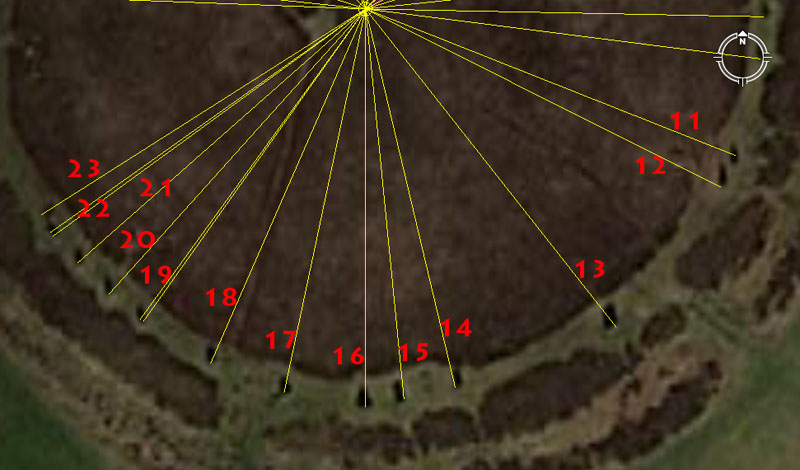11. This stone sits 172.8' out @ a return angle of 291.6-degrees.

The 291.6 value is navigational coding related to the 130636800' equatorial circumference (24883.2 Greek miles).

12. This stone sits 172.8' out @ 116.64-degrees.

There sum of 11664' would be 1/11200th of the 24883.2 Greek mile equatorial circumference of the Earth. The Roman foot was 11.664" and this value is navigational and lunar coding simultaneously.

13. This stone sits 176' out @ 141.75-degrees.

The sum of 176' would be 1/30th of a mile of 5280'.

The angle is part of a lunar progression and there would be 25 intervals of 14.175-days in a lunar year of 354.375-days. There would be 48 intervals of 141.75-days in the 6804-day lunar nutation cycle, whereas there would be 18 such intervals in the lunar period (2551.5-days ... 7.2 lunar years) of the lunisolar Sabbatical Calendar.

14. This stone sits 168.75' out @ 166.66666-degrees.

The sum of 16.875-days would be 1/21st of a lunar year.

15. This stone sits 170.1' out @ a return angle of 354.375-degrees.

The distance relates to the 6804-day lunar nutation cycle and the angle relates to the lunar year.

16. This stone sits 172.8' out @ due South of site centre (180-degrees).

Both the distance and angle offer dynamic navigational coding.

17. This stone sits 170.1-degrees out @ 192-degrees and a return angle of 12-degrees.

Mathematical progressions based upon 192 generated essential values used in navigational calculations. The sum of 12-degrees was 1/30th of a 360-degree circle.

18. This stone sits 168' out @ 23.625-degrees return.

A mathematical progression based upon 23.625 generates very important lunar and navigational numbers simultaneously.

19. This stone sits 166.66666' out @ a return angle of 35.4375-degrees and this alignment points directly at the trough between Ward Hill and Cuilags Hill of Hoy Island.

The value 166.66666' is navigational and is simply 1/3rd of 500'.

The return degree angle, in this instance, is very important and tells us why the centre of Brodgar Ring was sited in that exact position. The intention was to forever accentuate and encode the lunar year numerical value by making the return angle from Hoy Island's very conspicuous "V" trough read 35.4375-degrees (357/16ths). This means that the "V" trough at Hoy (SSE) was a permanent surveying outer marker, eternal benchmark or fix position from which Ring o' Brodgar was established and built. The lunar year is, of course, 354.375-days.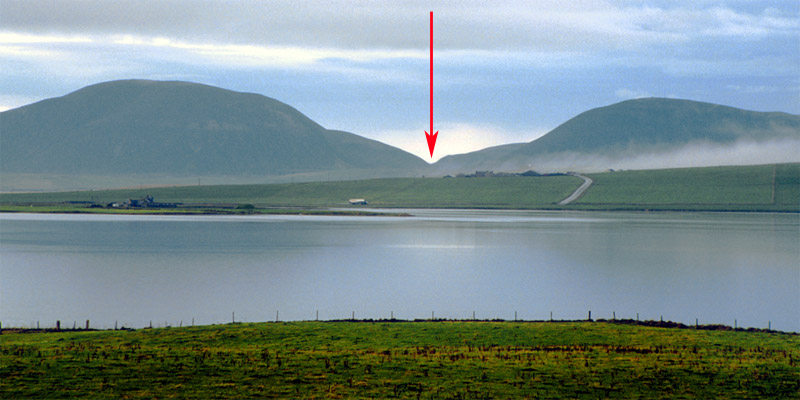The "V" at Hoy Island that acted as a permanent fix position or benchmark and as one of two major geological features that determined where the precise centre of Ring o' Brodgar had to be. The return angle from the trough of the "V" between Ward Hill (left) and Cuilags Hill (right) was 35.4375-degrees (357/16ths).

The "V" also determined exactly where Maes Howe chambered cairn was placed, with its doorway sitting 8 ancient Scottish miles (of 5940') or 9 English miles (of 5280') from the trough of the "V" on Hoy @ 224-degrees azimuth or 44-degrees return. From this very exact fix point on Hoy, ancient surveyors, setting up their manual theodolites atop Maes Howe cairn, could use the "V" fix point (at precisely 224-degrees azimuth) for setting their 360-degree calibrated disc. After that they could achieve perfect readings onto any other targets around the horizon. (Photo courtesy of: http://www.mrtattieheid.com/index.htm).

20. This stone sits 166.66666' out @ a return angle of 48.6-degrees.

The 48.6 value was very important to lunar calculations within the Sabbatical Calendar. The Roman mile @ 5000 Roman feet of 11.664" each was 4860' of 12" each.

21. This stone sits 168' out @ a return angle of 54-degrees.

The 54 value was very useful in calculating lunar periods, but was also a factorable number used in navigational progressions. The sum of 540' would be 1/11th of a Scottish mile or 1/9th of a Roman mile.

22. This stone sits 166.66666' out @ a return angle of 57.6-degrees.

The value 57.6 generates a very important mathematical progression related to navigation and the equatorial circumference of the Earth, as well as the duration of the Precession of the Equinoxes or the lunar nutation cycle, etc. It's a very versatile, much-used number in ancient calculations.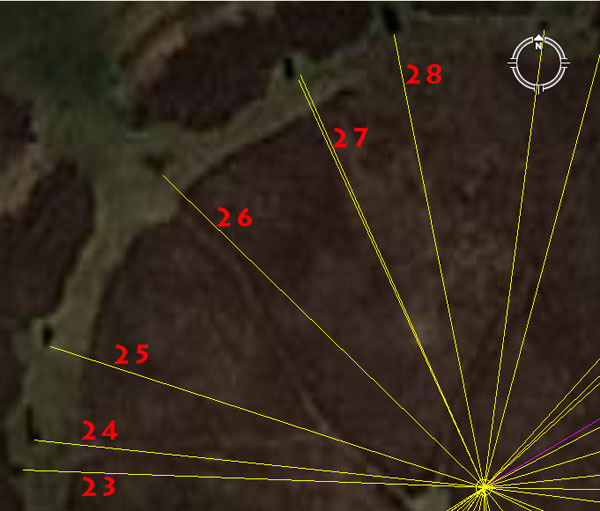23. This stone sits 170.1' out @ 92.16-degrees return.

The 92.16 value relates to the equatorial circumference of the Earth and there would be 27 segments of 921.6-miles in the 24883.2-mile circumference.

24. This stone sits 166.66666' out @ 96-degrees return.

The value 96 was used in navigational mathematical progressions and there would be 55 segments of 96' in an English mile (5280') or 70 segments in an Irish mile (6720'). There would be 27 occurrences of 960-years in the 25920-year duration of the Precession of the Equinoxes.

25. This stone sits 168' out @ 108-degrees return.

The 108 numerical progression is simply a doubled-out progression of the number 54 and very important to all manner of ancient cyclic or circumference calculations.

26. This stone sits 165' out @ 314.16-degrees.

The degree angle is, of course, indicating PI as an inflated expression (PI X 100). Tutorials related to the other forms of PI in constant usage for calculations within particular number families at this standing stone, such as 3.125, 1728/550ths (3.141818182), 22/7ths (3.142857143) or 3.15.

27. This stone sits 165' out @ a return angle of 155.52-degrees. It's very likely that another tutorial from this position related to an angle of 336-degrees.

The 155.52 value relates to the equatorial circumference of the Earth and the sum of 1555.2-miles would be 1/16th of the 24883.2-mile equatorial circumference.

The merits of the 336 value would also have been taught at this station. The sum of 3360' would be 1/2 of an Irish mile.

28. This stone sits 170.1' out @ a return angle of 168.75-degrees.

The 168.75 value is strong lunar coding and there would be 21 intervals of 16.875-days (405-hours) in a lunar year.

RING O' BRODGAR'S ADJACENT MOUNDS, CAIRNS AND THE COMET STONE.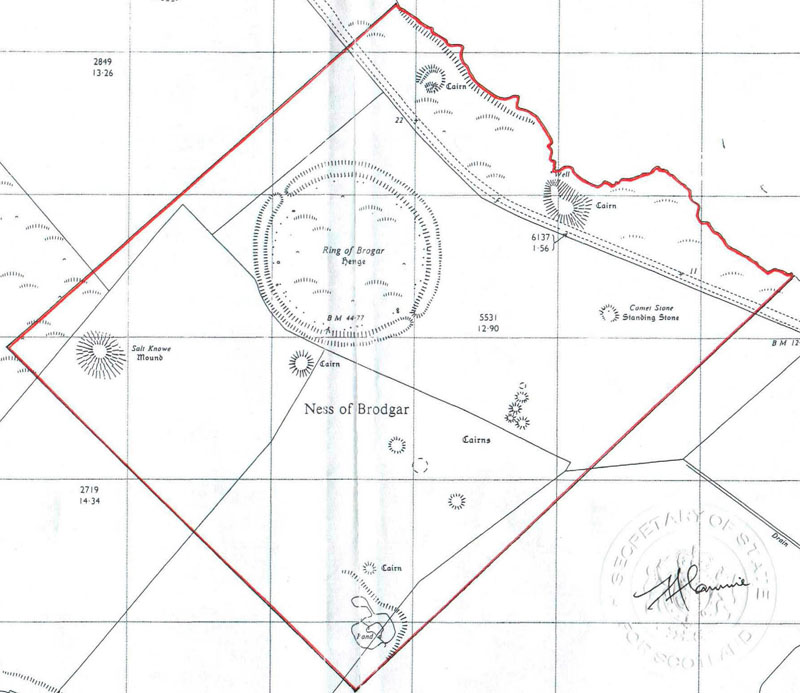In this official map-plan, Ring o' Brodgar is shown in conjunction with its surrounding satellite structures, including the large mound called Salt Knowe to the WSW.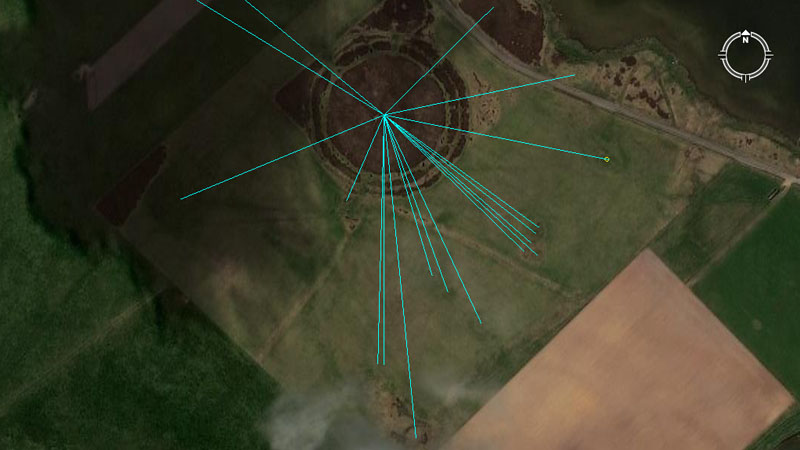Lines are seen to extend from the centre of Ring o' Brodgar to resolve on outlying structures in the immediate vicinity. Additional lines run off the picture to other structures some distance away to the NW.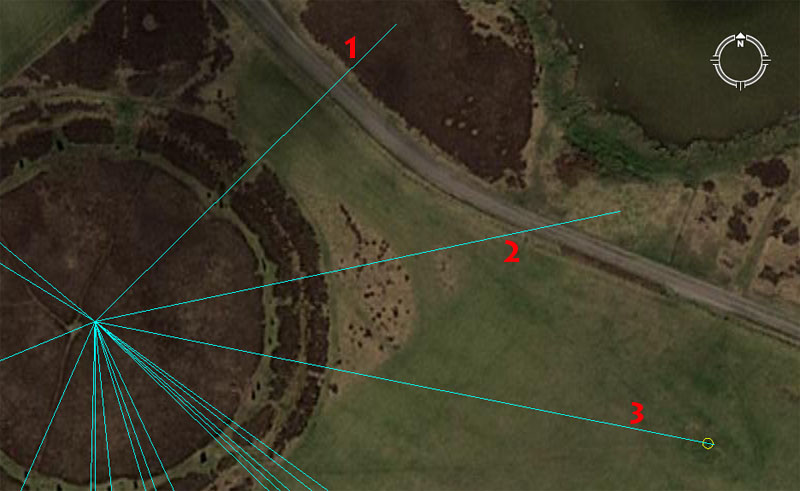Here are the apparent codes for these outlying structures.

1. The large cairn sits 414.72' out @ 45.36-degrees.

The 414.72 value relates to the equatorial circumference of the Earth and 4147.2-miles would be 1/6th of the 24883.2-mile circumference.

2. The large cairn sits 525' & 528' out @ 78.125-degrees.

This mound would have been used to teach the merits of two forms of miles (5250' ... "6&7" number families combined and 5280' ... "11" number family).

The degree angle value generates a mathematical progression used in navigation and lunar calculations.

3. The Comet Stone sits 611.111' (6111/9th) out @ 101.25-degrees, but one of the main codes for this mound (with its additional stone bases still in place) is 618.034'.

The distance of 611.11111' is simply 1/9th of 5500' (1000 ancient fathoms).

There are, however, additional stone stumps on this mound and it's probable that one related to the much used and highly venerated PHI reciprocal. The distance, in that case, would have been read as 618.034' and be used as a mnemonic device to remember 6.18034", an increment that was highly important to fabricating precise volume vessels for the marketplace (amongst all the cousin nations). The increment is based upon the formula, 10'' ÷ PHI (1.6180339) = 6.18034".

The degree angle is strong lunar coding and there would be 35 intervals of 10.125-days (243-hours) in a lunar year. There would be 252 intervals of 10.125-days in the 2551.5-day lunar period monitored within the Sabbatical Calendar and 672 such intervals in the 6804-day duration of the lunar nutation cycle.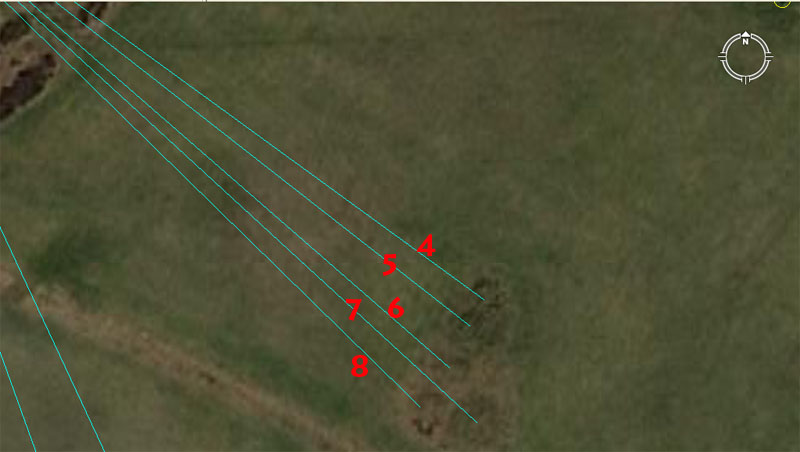Here are the apparent codes for the cairn cluster.

4. This cairn sits 515.625' out @ 126-degrees.

The distance is 300 Egyptian Royal cubits of 20.626" each (this was supposed to be the length of Noah's Arc ... if the "cubit" referred to in the Biblical account were Egyptian Royal Cubits. There were, of course, two other variations @ 20.61818182" and 20.736"). The sum of 515.625-miles (of 5280' each) would equate to 1/48th of the 24750-mile equatorial circumference of the Earth ("11" navigation system).

At the same time, the sum of 24883.2 Greek miles of 5250' each equates to 24741.81818181 English miles of 5280' and if this distance out from the centre of Ring o' Brodgar was read according to the "6&7" Egyptian Royal Cubit of 20.61818182" (an example of one of these is found in the Turin Museum) then that reading in miles would also be 1/48th of the equatorial circumference of the Earth.

The value 126 is one of the most important of antiquity and the "6&7" measurement was founded on this increment. The Greek foot was 12.6"; the Hebrew reed was 126" (10.5').

5. This cairn sits 518.4' out @ 128-degrees.

This distance is 300 of the largest Egyptian Royal Cubits @ 20.736" (1.728'). Although it doesn't appear to have been used as a navigational system, the "true" size of the Earth was described in factorable numbers as 24883.2 miles of 5280' (only 18.8-miles short of the value we use today). Therefore 518.4 miles (of 5280' each) would be 1/48th of the equatorial circumference under the "true" reading.

The value 128 sets up a very important numerical progression used in navigation. The ancient French had a foot of 12.8" (1.066666' ordinary feet of 12"). The large brass panel, Calendar of Coligny artefact (Galois) is made up of 128 squares.

6. This cairn sits 525' out @ a return angle of 311.04-degrees.

This distance is 1 Greek short stadia or 1/10th of a Greek mile.

The 311.04 value is very important navigational coding related to the equatorial circumference of the Earth and 3110.4-miles would be 1/8th of the 24883.2-mile equatorial circumference.

7. This cairn sits 560' out @ a return angle of 312.5-degrees.

The 560' distance would be 1/12th of an Irish mile (6720'). The value 56 was used in mathematical progressions to generate many useful navigational and lunar cycle numbers.

The degree angle is, of course the dissecting angle through the Avenue of Ring o' Brodgar and also the angle one had to use to get to Iceland when leaving Scapa Flow between Hoy-Mainland Islands and traveling NW. There would be 3.125 miles in a league.

8. This cairn sits 528' out @ 314.2857143-degrees.

The 528' value is 1/10th of a mile of 5280'.

The degree angle codes PI X 100 using 22/7 to generate the value. This same position would have offered a similar tutorial for 1728/550.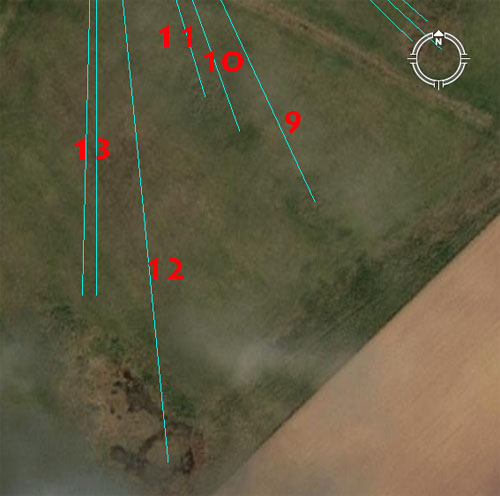Here are the apparent codes for the structures to the immediate South of Ring o' Brodgar.

9. This cairn sits 618.034' out @ a return angle of 336-degrees. A tutorial related to an angle of 155.52-degrees would also have been encoded into this position.

The distance tutorial relates to the PHI reciprocal, which had to be memorised in order that round tubs, with perfect, whole, factorable square inch values for their bases could be fabricated for the marketplace. After that it was a very simple matter to make the sides of the tubs a code-bearing height in order to get a perfectly coded cubic capacity that related to navigation, the lunar cycle or some other value from the parcel of special scientific numbers. The area within a circle of 618.034' diameter would be 300,000 sq. ft.

The angle value of 336-degrees codes a relationship to the Irish mile and 336' is 1/20th of an Irish mile of 6720'.

within the dimensions of the cairn a vector of 155.52-degrees return would also have applied and 1555.2-miles is 1/16th of the 24883.2-mile equatorial circumference. The angle value and what it generated in a mathematical progression would have been taught in relation to this cairn position.

10. This cairn sits 506.25' out @ a return angle of 340.2-degrees.

The 506.25' value is lunar coding and there would be 7 intervals of 50.625-days (1215 hours) in the lunar year.

The angle of 304.2-degrees is also lunar and relates to the 6804-day lunar nutation cycle. Ring o' Brodgar is 304.2' across, as is the southern circle of Avebury Henge.

11. This cairn sits 453.6' out @ 163.35-degrees.

The vertical height of the Great Pyramid of Egypt, from the desert base to the top of its flat altar floor is 453.6' (it never had a capstone and was built as a truncated pyramid with a top altar). The coding relates to the equatorial circumference of the Earth and 453600 feet would be 1/288th of the 24883.2 Greek mile equatorial circumference.

Similarly, the sum of 16335' would be 1/8000th of the 24750-mile (of 5280') equatorial circumference.

12. This cairn sits 875 feet out @ a return angle of 354.375-degrees.

The 875 value sets up a mathematical progression that was very important to both navigation and the lunar cycles. Also, under the Sabbatical Calendar system there were 8.75 days of error accrued at the end of 7-solar years, which were corrected and added in as intercalary days in a festival lasting 9-days.

The return angle simply related to the lunar year (354.375-days).

13. This cairn sits 672' out @ 180-degrees. Another angle code that would have been taught from this position is 181.44-degrees.

This distance is 1/10th of an Irish mile.

The angle is due South. It's conceivable that the value 181.44 would have been taught from this same cairn position as it relates to the equatorial size of the Earth in minutes or degrees of arc. One circumnavigation of the Great Pyramid (1/2 a minute of arc) is 36288" (18144 X 2). Similarly, 1-degree of arc for the Earth is 362880' (181440 X 2).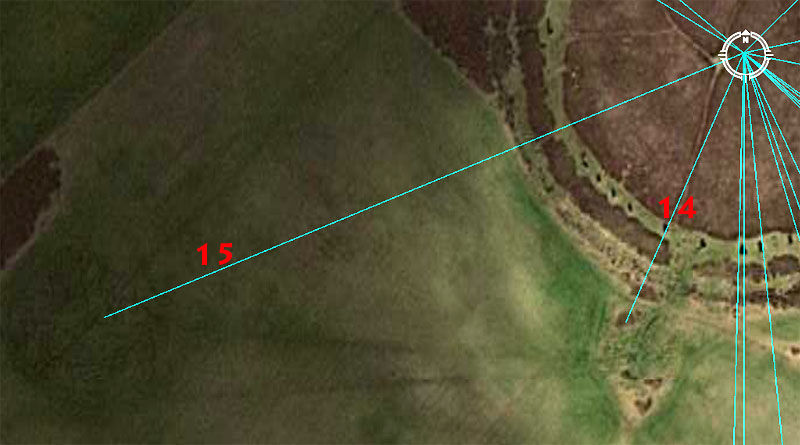Here is the code for Salt Knowe Mound, as well as a cairn at the South side of Ring o' Brodgar.

14. This cairn sits 252' out @ a return angle of 23.625-degrees.

The sum of 252' would be 1/3rd of the length of the Great Pyramid or 1/24th of 1-minute of the Earth's equatorial arc. The length would also equate to 240 Greek feet of 12.6" each, 120 Assyrian cubits or 24 Hebrew reeds.

The degree angle is strong lunar coding and 23.625-days (235/8ths) would be 1/15th of a lunar year.

15. Salt Knowe Mound sits 594' out @ 247.5-degrees.

The distance to the crown of Salt Knowe coded 1/10th of a Scottish mile of 5940'.

The angle code is in homage to the 24750-mile (of 5280') equatorial circumference of the Earth (which also equates to 22000 Scottish miles).